# PSF Matching (photutils.psf.matching)¶

## Introduction¶

This subpackage contains tools to generate kernels for matching point spread functions (PSFs).

## Matching PSFs¶

Photutils provides a function called create_matching_kernel() that generates a matching kernel between two PSFs using the ratio of Fourier transforms (see e.g., Gordon et al. 2008; Aniano et al. 2011).

For this first simple example, let’s assume our source and target PSFs are noiseless 2D Gaussians. The “high-resolution” PSF will be a Gaussian with $$\sigma=3$$. The “low-resolution” PSF will be a Gaussian with $$\sigma=5$$:

>>> import numpy as np
>>> from astropy.modeling.models import Gaussian2D
>>> y, x = np.mgrid[0:51, 0:51]
>>> gm1 = Gaussian2D(100, 25, 25, 3, 3)
>>> gm2 = Gaussian2D(100, 25, 25, 5, 5)
>>> g1 = gm1(x, y)
>>> g2 = gm2(x, y)
>>> g1 /= g1.sum()
>>> g2 /= g2.sum()


For these 2D Gaussians, the matching kernel should be a 2D Gaussian with $$\sigma=4$$ (sqrt(5**2 - 3**2)). Let’s create the matching kernel using a Fourier ratio method. Note that the input source and target PSFs must have the same shape and pixel scale.

>>> from photutils import create_matching_kernel
>>> kernel = create_matching_kernel(g1, g2)


Let’s plot the result:

import numpy as np
from astropy.modeling.models import Gaussian2D
from photutils import create_matching_kernel
import matplotlib.pyplot as plt

y, x = np.mgrid[0:51, 0:51]
gm1 = Gaussian2D(100, 25, 25, 3, 3)
gm2 = Gaussian2D(100, 25, 25, 5, 5)
g1 = gm1(x, y)
g2 = gm2(x, y)
g1 /= g1.sum()
g2 /= g2.sum()

kernel = create_matching_kernel(g1, g2)
plt.imshow(kernel, cmap='Greys_r', origin='lower')
plt.colorbar()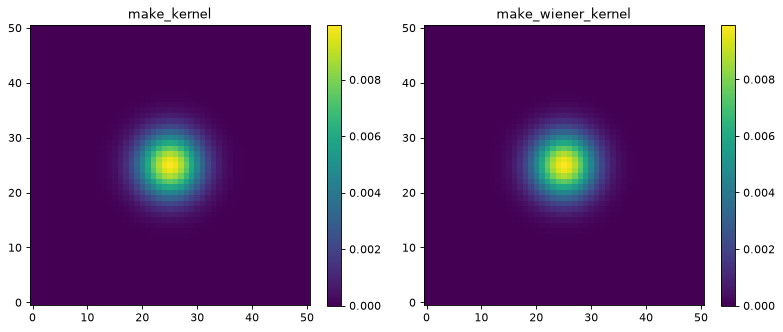We quickly observe that the result is not as expected. This is because of high-frequency noise in the Fourier transforms (even though these are noiseless PSFs, there is floating-point noise in the ratios). Using the Fourier ratio method, one must filter the high-frequency noise from the Fourier ratios. This is performed by inputing a window function, which may be a function or a callable object. In general, the user will need to exercise some care when defining a window function. For more information, please see Aniano et al. 2011.

Photutils provides the following window classes:

Here are plots of 1D cuts across the center of the 2D window functions:

from photutils import (HanningWindow, TukeyWindow, CosineBellWindow,
SplitCosineBellWindow, TopHatWindow)
import matplotlib.pyplot as plt
w1 = HanningWindow()
w2 = TukeyWindow(alpha=0.5)
w3 = CosineBellWindow(alpha=0.5)
w4 = SplitCosineBellWindow(alpha=0.4, beta=0.3)
w5 = TopHatWindow(beta=0.4)
shape = (101, 101)
y0 = (shape - 1) // 2

plt.figure()

plt.subplot(2, 3, 1)
plt.plot(w1(shape)[y0, :])
plt.title('Hanning')
plt.xlabel('x')
plt.ylim((0, 1.1))

plt.subplot(2, 3, 2)
plt.plot(w2(shape)[y0, :])
plt.title('Tukey')
plt.xlabel('x')
plt.ylim((0, 1.1))

plt.subplot(2, 3, 3)
plt.plot(w3(shape)[y0, :])
plt.title('Cosine Bell')
plt.xlabel('x')
plt.ylim((0, 1.1))

plt.subplot(2, 3, 4)
plt.plot(w4(shape)[y0, :])
plt.title('Split Cosine Bell')
plt.xlabel('x')
plt.ylim((0, 1.1))

plt.subplot(2, 3, 5)
plt.plot(w5(shape)[y0, :], label='Top Hat')
plt.title('Top Hat')
plt.xlabel('x')
plt.ylim((0, 1.1))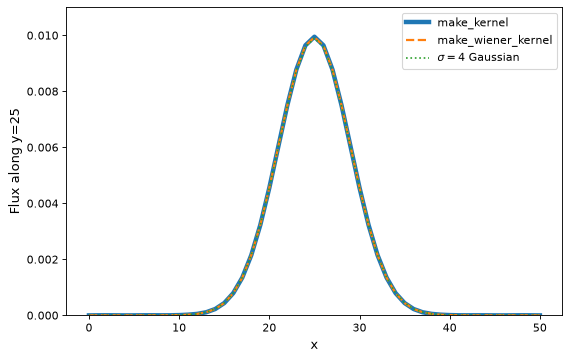However, the user may input any function or callable object to generate a custom window function.

In this example, because these are noiseless PSFs, we will use a TopHatWindow object as the low-pass filter:

>>> from photutils import TopHatWindow
>>> window = TopHatWindow(0.35)
>>> kernel = create_matching_kernel(g1, g2, window=window)


Note that the output matching kernel from create_matching_kernel() is always normalized such that the kernel array sums to 1:

>>> print(kernel.sum())
1.0


Let’s display the new matching kernel:

import numpy as np
from astropy.modeling.models import Gaussian2D
from photutils import create_matching_kernel, TopHatWindow
import matplotlib.pyplot as plt

y, x = np.mgrid[0:51, 0:51]
gm1 = Gaussian2D(100, 25, 25, 3, 3)
gm2 = Gaussian2D(100, 25, 25, 5, 5)
g1 = gm1(x, y)
g2 = gm2(x, y)
g1 /= g1.sum()
g2 /= g2.sum()

window = TopHatWindow(0.35)
kernel = create_matching_kernel(g1, g2, window=window)
plt.imshow(kernel, cmap='Greys_r', origin='lower')
plt.colorbar()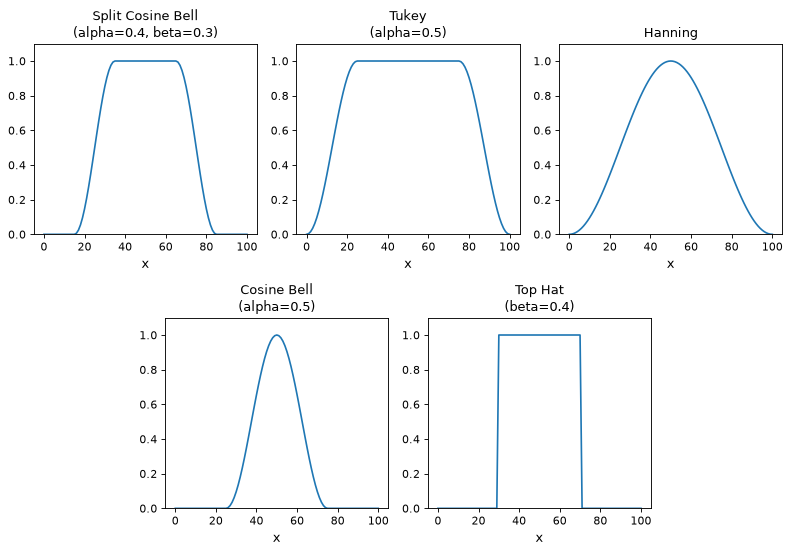As desired, the result is indeed a 2D Gaussian with a $$\sigma=4$$. Here we will show 1D cuts across the center of the kernel images:

import numpy as np
from astropy.modeling.models import Gaussian2D
from photutils import create_matching_kernel, TopHatWindow
import matplotlib.pyplot as plt

y, x = np.mgrid[0:51, 0:51]
gm1 = Gaussian2D(100, 25, 25, 3, 3)
gm2 = Gaussian2D(100, 25, 25, 5, 5)
gm3 = Gaussian2D(100, 25, 25, 4, 4)
g1 = gm1(x, y)
g2 = gm2(x, y)
g3 = gm3(x, y)
g1 /= g1.sum()
g2 /= g2.sum()
g3 /= g3.sum()

window = TopHatWindow(0.35)
kernel = create_matching_kernel(g1, g2, window=window)
kernel /= kernel.sum()
plt.plot(kernel[25, :], label='Matching kernel')
plt.plot(g3[25, :], label='$\sigma=4$ Gaussian')
plt.xlabel('x')
plt.ylabel('Flux')
plt.legend()
plt.ylim((0.0, 0.011))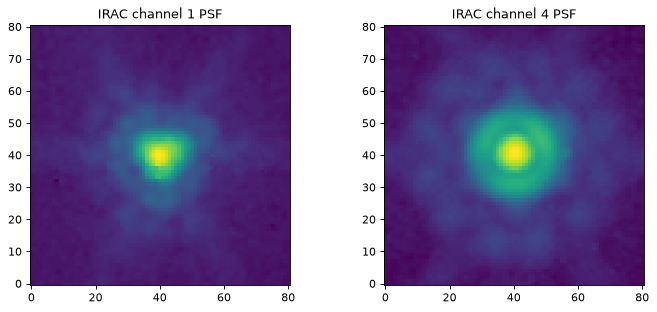## Matching IRAC PSFs¶

For this example, let’s generate a matching kernel to go from the Spitzer/IRAC channel 1 (3.6 microns) PSF to the channel 4 (8.0 microns) PSF. We load the PSFs using the load_irac_psf() convenience function:

>>> from photutils.datasets import load_irac_psf
>>> ch1 = ch1_hdu.data
>>> ch4 = ch4_hdu.data


Let’s display the images:

import matplotlib.pyplot as plt
from astropy.visualization import LogStretch
from astropy.visualization.mpl_normalize import ImageNormalize

ch1 = ch1_hdu.data
ch4 = ch4_hdu.data
norm = ImageNormalize(stretch=LogStretch())

plt.figure(figsize=(9, 4))

plt.subplot(1, 2, 1)
plt.imshow(ch1, norm=norm, cmap='viridis', origin='lower')
plt.title('IRAC channel 1 PSF')

plt.subplot(1, 2, 2)
plt.imshow(ch4, norm=norm, cmap='viridis', origin='lower')
plt.title('IRAC channel 4 PSF')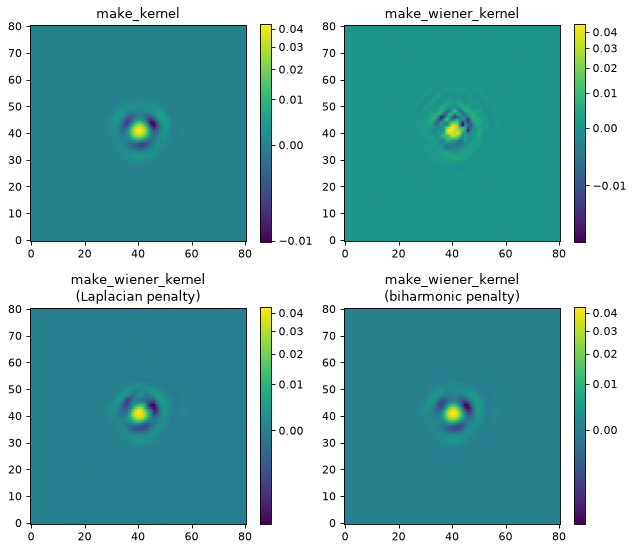For this example, we will use the CosineBellWindow for the low-pass window. Note that these Spitzer/IRAC channel 1 and 4 PSFs have the same shape and pixel scale. If that is not the case, one can use the resize_psf() convenience function to resize a PSF image. Typically, one would interpolate the lower-resolution PSF to the same size as the higher-resolution PSF.

>>> from photutils import CosineBellWindow, create_matching_kernel
>>> window = CosineBellWindow(alpha=0.35)
>>> kernel = create_matching_kernel(ch1, ch4, window=window)


Let’s display the matching kernel result:

import matplotlib.pyplot as plt
from astropy.visualization import LogStretch
from astropy.visualization.mpl_normalize import ImageNormalize
from photutils import CosineBellWindow, create_matching_kernel

ch1 = ch1_hdu.data
ch4 = ch4_hdu.data
norm = ImageNormalize(stretch=LogStretch())

window = CosineBellWindow(alpha=0.35)
kernel = create_matching_kernel(ch1, ch4, window=window)

plt.imshow(kernel, norm=norm, cmap='viridis', origin='lower')
plt.colorbar()
plt.title('Matching kernel')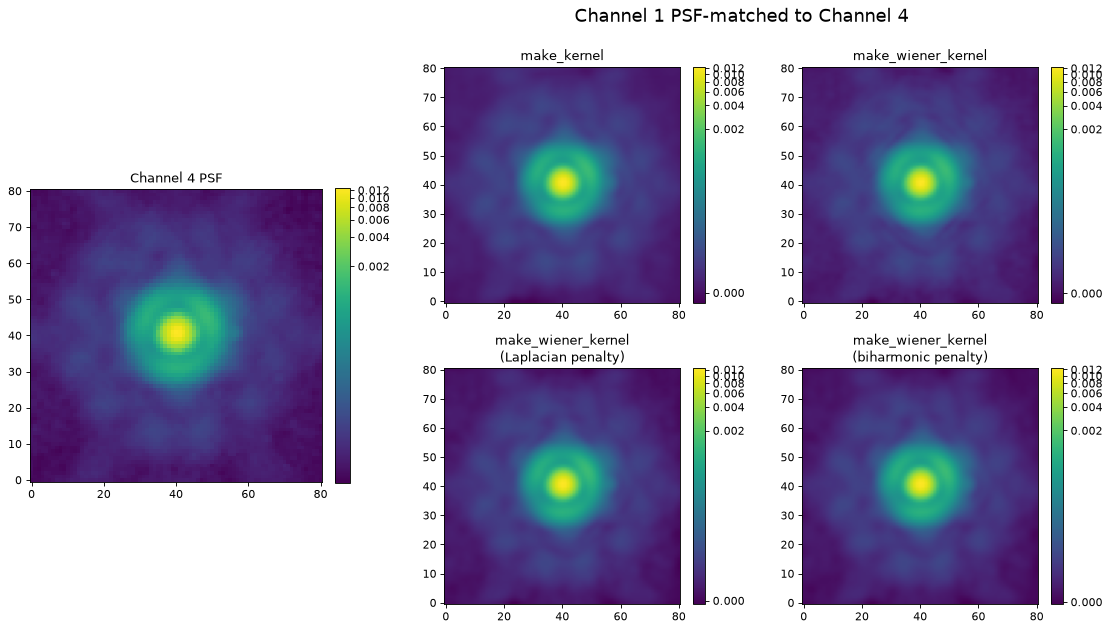The Spitzer/IRAC channel 1 image could then be convolved with this matching kernel to produce an image with the same resolution as the channel 4 image.

## Reference/API¶

This subpackage contains tools to generate kernels for matching point spread functions.

### Functions¶

 create_matching_kernel(source_psf, target_psf) Create a kernel to match 2D point spread functions (PSF) using the ratio of Fourier transforms. resize_psf(psf, input_pixel_scale, …[, order]) Resize a PSF using spline interpolation of the requested order.

### Classes¶

 CosineBellWindow(alpha) Class to define a 2D cosine bell window function. Class to define a 2D Hanning (or Hann) window function. SplitCosineBellWindow(alpha, beta) Class to define a 2D split cosine bell taper function. TopHatWindow(beta) Class to define a 2D top hat window function. TukeyWindow(alpha) Class to define a 2D Tukey window function.